Question

The figure shows a circuit with an ideal battery and set of capacitors. The battery has an emf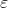=12 V. The capacitors have capacitances C1 = C2 = C3 = C4 = 6 nF.

(a) What is the equivalent capacitance of the entire network of capacitors?

(b) What is the electrical energy stored in the capacitor with capacitance C2?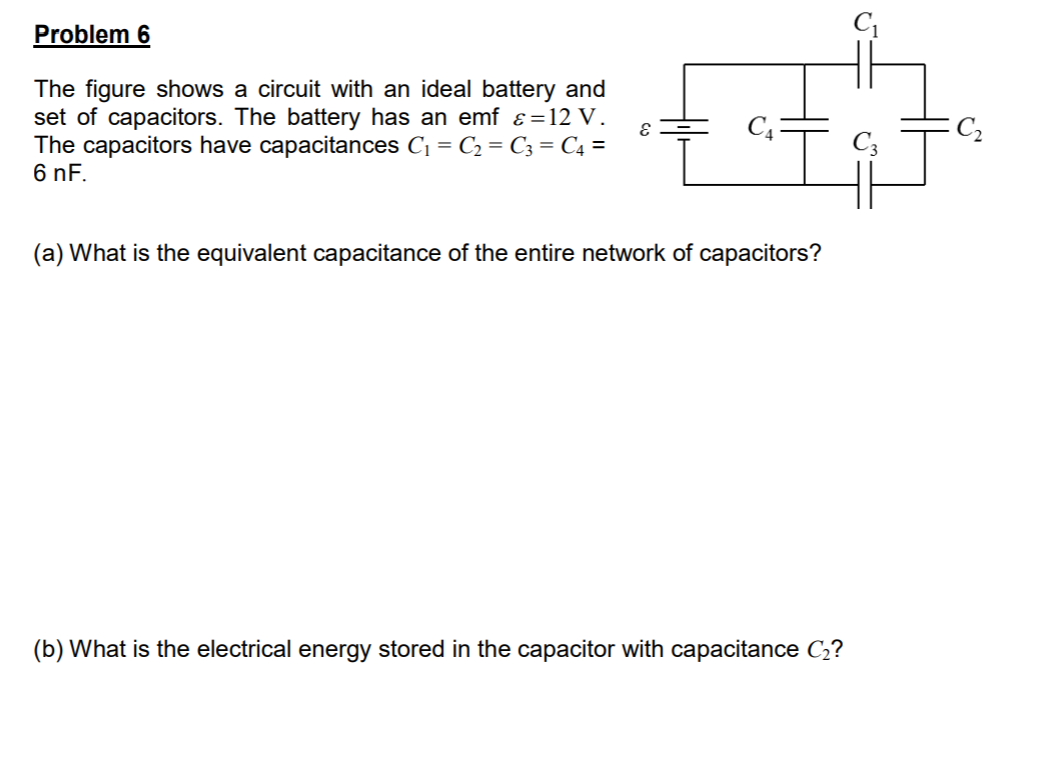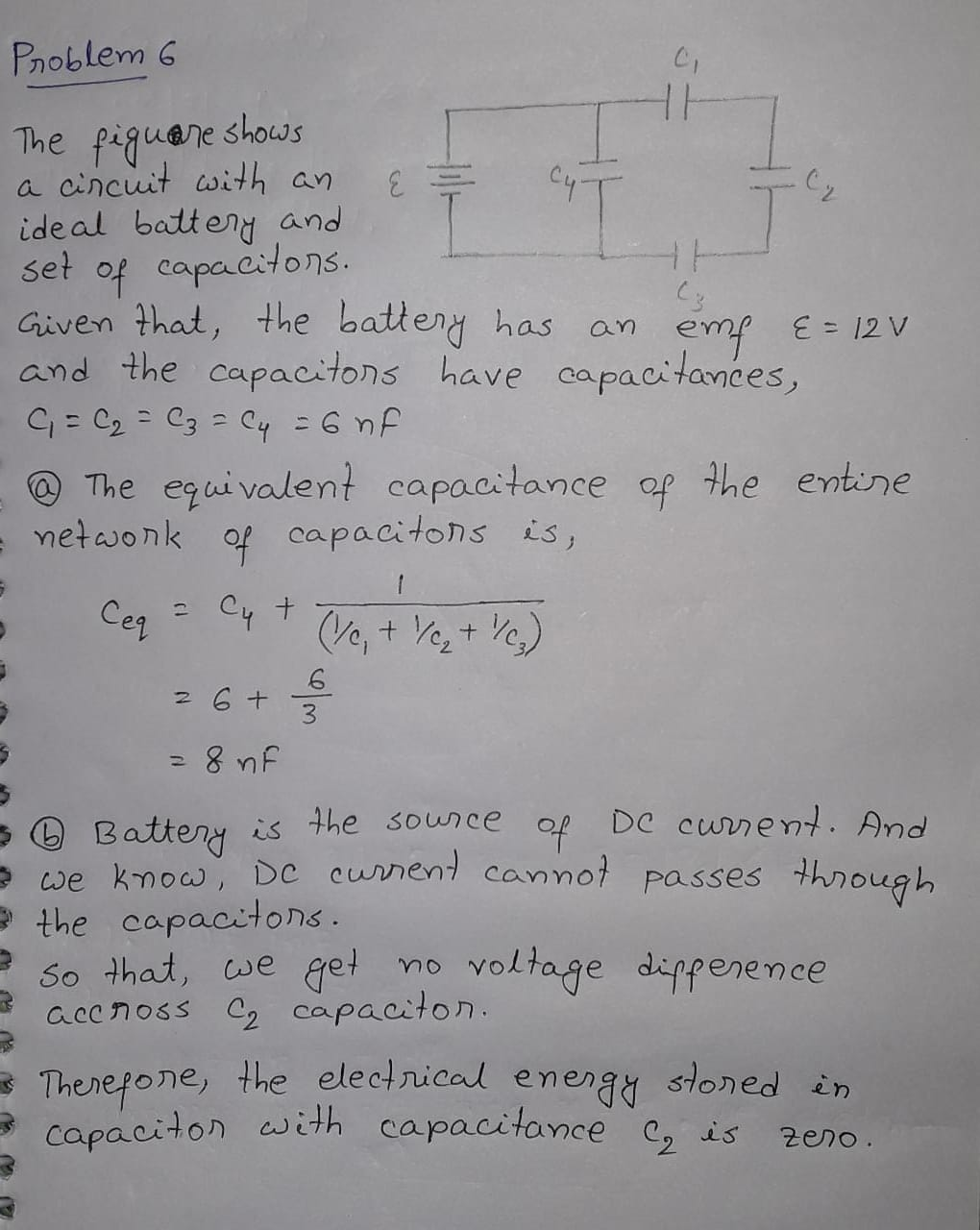#### Earn Coins

Coins can be redeemed for fabulous gifts.

Similar Homework Help Questions
• ### Four capacitors are arranged in the circuit shown in the figure. The capacitors have the values...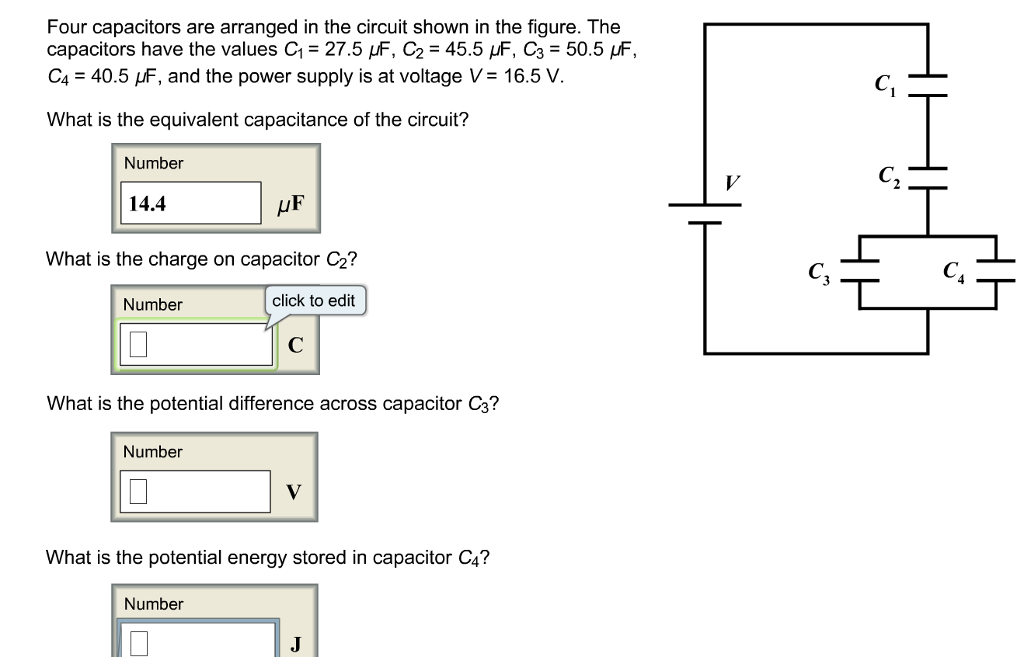Four capacitors are arranged in the circuit shown in the figure. The capacitors have the values C1 = 27.5 μF, C2 = 45.5 μF, C3 = 50.5 μF, C4 = 40.5 μF, and the power supply is at voltage V = 16.5 V. What is the equivalent capacitance of the circuit? Four capacitors are arranged in the circuit shown in the figure. The capacitors have the values C1-27.5 μF, C2 = 45.5 μF, C3 = 50.5 F, C.-40.5 and the...

• ### The circuit in the figure below contains a 90.0 V battery and four capacitors. In the...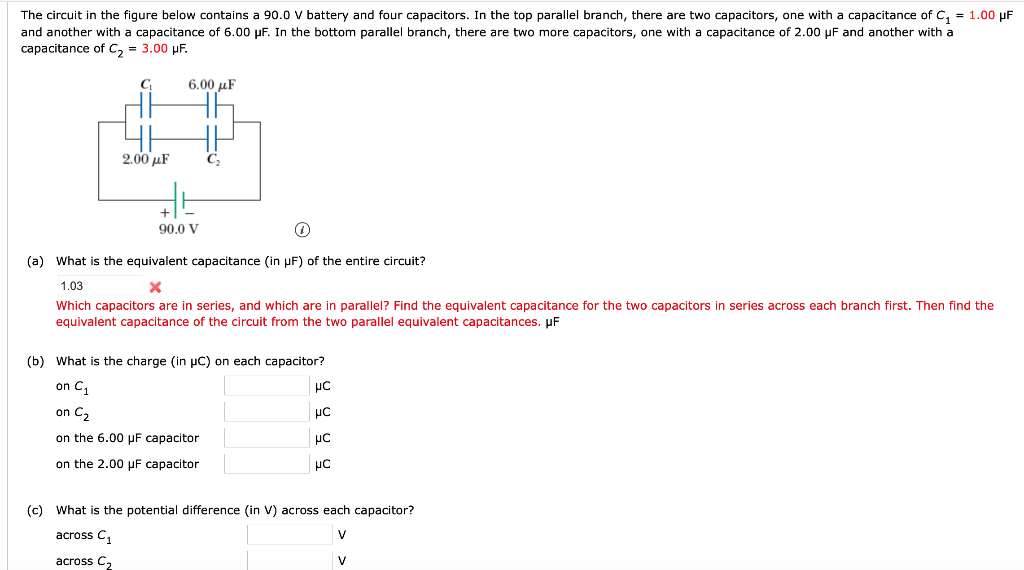The circuit in the figure below contains a 90.0 V battery and four capacitors. In the top parallel branch, there are two capacitors, one with a capacitance of C = 1.00 pF and another with a capacitance of 6.00 pF. In the bottom parallel branch, there are two more capacitors, one with a capacitance of 2.00 pF and another with a capacitance of C2 = 3.00 uF. C 6.00 uF 2.00 uF 90.0 V (a) What is the equivalent capacitance...

• ### In the circuit shown in the figure the voltage of the battery is 15.0 V, and...In the circuit shown in the figure the voltage of the battery is 15.0 V, and the capacitors have the following capacitances: C1 = 1.86 mF, C2 = 3.39 mF, C3 = 3.85 mF, C4 = 5.79 mF. What is the equivalent capacitance of the four capacitors?

• ### A circuit is constructed with five capacitors and a battery as shown. The values for the...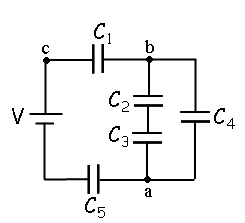A circuit is constructed with five capacitors and a battery as shown. The values for the capacitors are: C1 = C5 = 4.2 μF, C2 = 1 μF, C3 = 5.8 μF, and C4 = 4.4 μF. The battery voltage is V = 12 V. 1) What is Cab, the equivalent capacitance between points a and b?μF 2) What is Cac, the equivalent capacitance between points a and c? 3) What is Q5, the charge on capacitor C5?μC 4) What...

• ### Q22 (Capacitors) The figure below shows a 15.0 V battery and four uncharged capacitors of capacitances...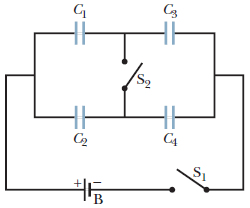Q22 (Capacitors) The figure below shows a 15.0 V battery and four uncharged capacitors of capacitances C1 = 8.00 μF, C2 = 4.00 μF, C3 = 6.00 μF, and C4 = 3.00 μF. If only switch S1 is closed, what is the charge on each capacitor? (a)    C1 __________ μC (b)    C2 __________ μC (c)    C3 __________μC (d)    C4 __________ μC If both switches are closed, what is the charge on each capacitor? (e)    C1 __________μC (f )    C2 __________μC (g)    C3 __________ μC (h)    C4 __________ μC...

• ### A circuit is constructed with five capacitors and a battery as shown. The values for...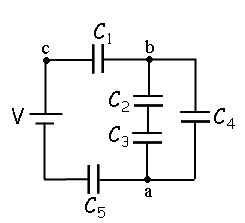A circuit is constructed with five capacitors and a battery as shown. The values for the capacitors are: C1 = C5 = 3.9 μF, C2 = 1.9 μF, C3 = 7.6 μF, and C4 = 2.2 μF. The battery voltage is V = 12 V. 4)What is Q2, the charge on C2? 5)What is Q1, the charge on capacitor C1? C1 b HH Cz C4 Ca HH a C5

• ### Two capacitors, C119.0 F and C2 32.0 uf are connected in series, and a 9.0-V battery...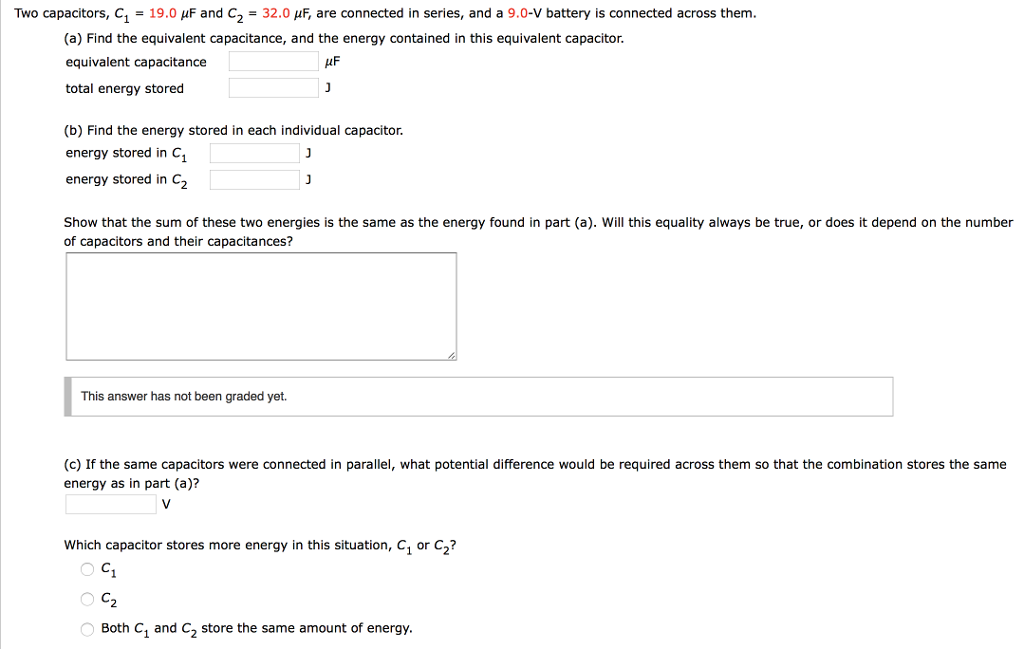Two capacitors, C119.0 F and C2 32.0 uf are connected in series, and a 9.0-V battery is connected across them (a) Find the equivalent capacitance, and the energy contained in this equivalent capacitor. equivalent capacitance total energy stored (b) Find the energy stored in each individual capacitor. energy stored in C1 energy stored in C2 Show that the sum of these two energies is the same as the energy found in part (a). Will this equality always be true, or...

• ### The circuit in the figure below shows four capacitors connected to a battery. The switch s...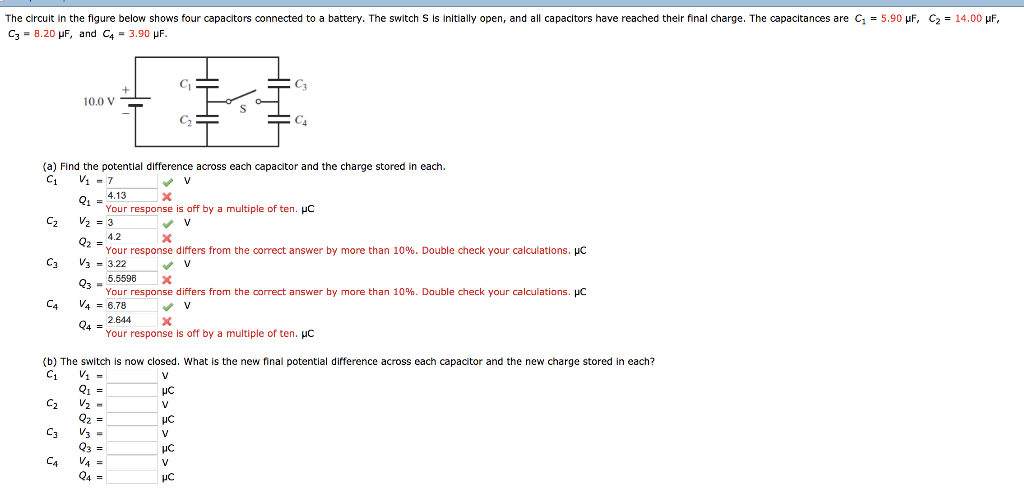The circuit in the figure below shows four capacitors connected to a battery. The switch s is initially open, and all capacitors have reached their final charge. The capacitances are C1 = 5.90 μF, C3 = 8.20 μF, and C4 = 3.90 μF C2 = 14.00 μF 10.0 V (a) Find the potential difference across each capacitor and the charge stored in each. 4.13 Your response is off by a multiple of ten. HC 4.2 Your response differs from the...

• ### 20 of 22 > Four capacitors are arranged in the circuit shown in the figure. The...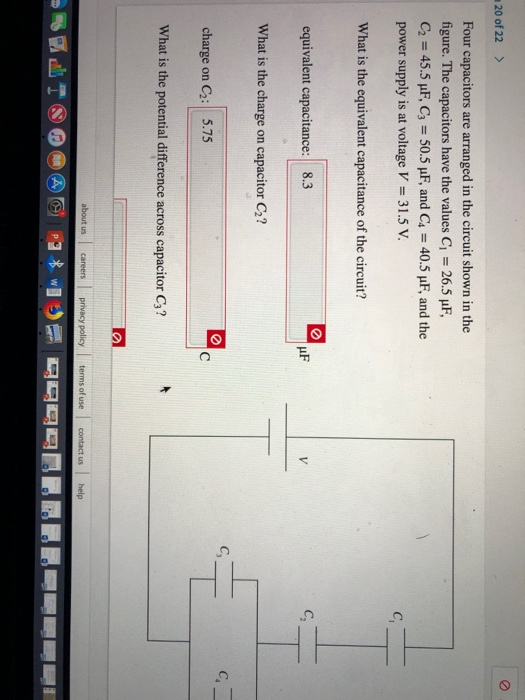20 of 22 > Four capacitors are arranged in the circuit shown in the figure. The capacitors have the values C 26.5 uF, C2 45.5 uF, C3 50.5 pF, and C4 40.5 uF, and the power supply is at voltage V 31.5 V C What is the equivalent capacitance of the circuit? C equivalent capacitance: 8.3 V What is the charge capacitor C2? on C. charge C2: 5.75 C on What is the potential difference across capacitor C3? about us...

• ### The circuit in the figure below contains a 9.00 v battery and four capacitors. The two...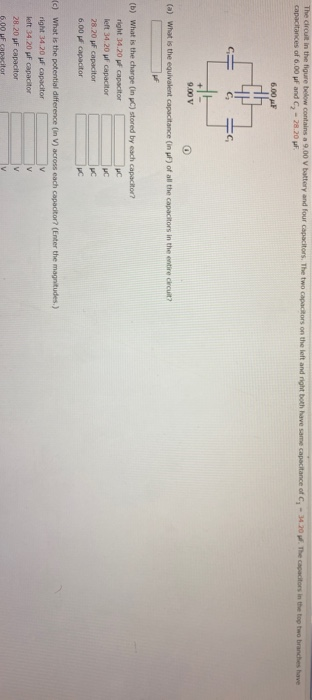The circuit in the figure below contains a 9.00 v battery and four capacitors. The two capacitors on the left and right both have same capacitance of C, -14.20. The capacitors in the top two branches have capacitances of 6.00 pF and C - 28.20 F 6.00 с. 9.00 V (a) What is the equivalent capacitance (in pf) of all the capacitors in the entire circuit? (b) What is the charge (in) stored by each capacitor? right 34.20 pf capacitor...